# Research

I defended a PhD in Computer Science in November 2016. I work on cryptography, which intersects mathematics, computer science, and electrical engineering. My research topic is at the boundary of the first two disciplines.

Key words: crypatanalysis, public key cryptography, discrete logarithm, finite fields, number field sieve, elliptic curve signatures (ECDSA), side channel attacks, bounded distance decoding problem (BDD), public randomness generation, blockchains...

## Discrete logarithms

Cryptography is the study of techniques for secure communication in the presence of third parties, also called adversaries. Such techniques are detailed in cryptosystems, explaining how to securely encode and decode messages. They are designed around computational hardness assumptions related to mathematical properties, making such algorithms hard to break in practice by any adversary. These protocols are based on the computational difficulty of various problems which often come from number theory, such as integer factorization or discrete logarithms computations. My research topic is the study of discrete logarithm algorithms in finite fields. The uninitiated lector is kindly invited to read an introduction of the discrete logarithm problem (DLP) here.

### Medium and High Characteristics

Insiders could find in the Special Number Field Sieve an algorithm to solve the DLP in medium and high characteristic finite field related to pairing-based cryptography whereas the Multiple Number Field Sieve works in the general medium and high characteristic cases. A new variant of NFS has been proposed by collegues in medium characteristic and is based on a polynomial selection with conjugation method. Combining these two variants, the Multiple Number Field Sieve with Conjugation Method is currently the best algorithm for all medium characteristic finite fields.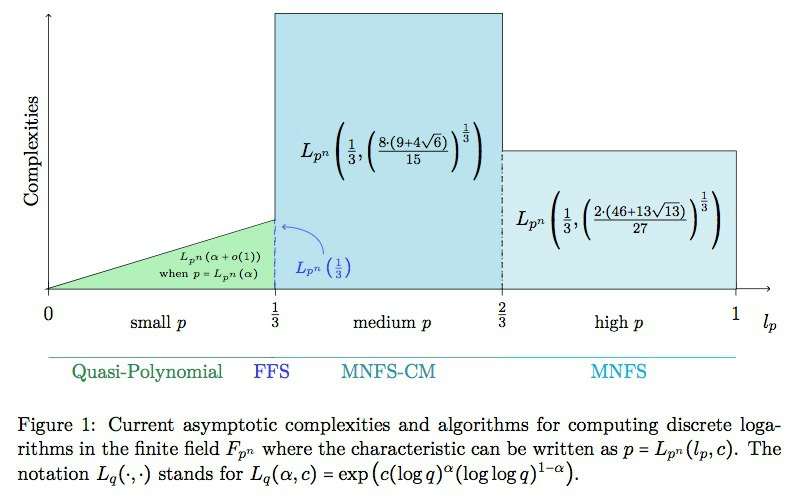### Small characteristic

The Simplified Frobenius Representation Algorithm permits to solve the DLP in small characteristic finite fields. It decreases the asymptotic complexities of the first polynomial phases, namely the ones that recover the discrete logarithms of the factor base elements (and of the extended factor base as well). Even if they are asymptotically negligible with regards to the quasi-polynomial individual logarithm phase, they are, in practice, the major brake on discrete logarithms computations. We illustrate the complexity lowering from O(q^7) to O(q^6) in the general case with a discrete logarithm record in characteristic 3. The target finite field consists in the extension field of degree 479 over the base field with 3^5 elements. The order of the target field is so a 3796-bits integer - to be compared with the previous record on characteristic 3 concerning a 1551-bits field.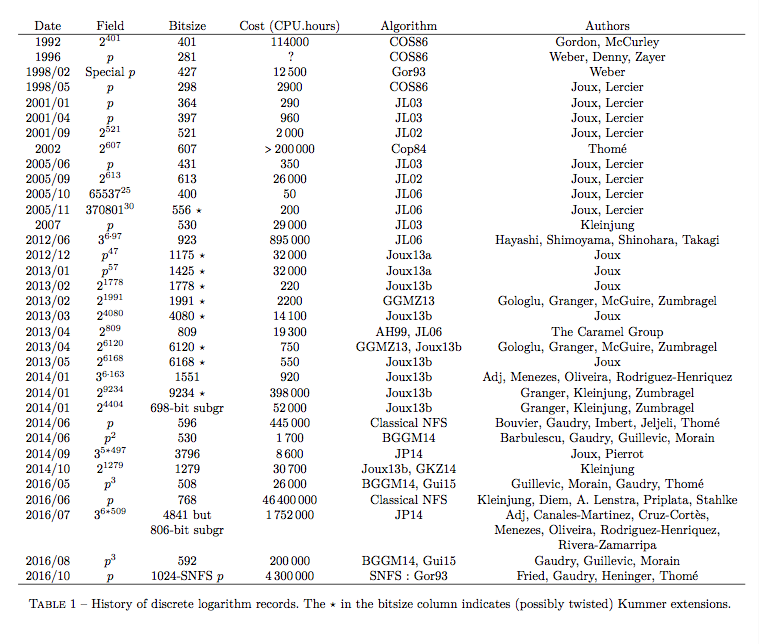### At the boundary between small and medium caracteristics

Finite fields used in pairing-based cryptography asymptotically live at this boundary case. However the discrete logarithm problem is very particular here, since it's the boundary of all relevant families of algorithms: quasipolynomial algorithms, and its ancestor the function field sieve (FFS), together with the number field sieve (NFS) and all its variants. In Asymptotic complexities of discrete logarithm algorithms in pairing-relevant finite fields, we adapt FFS to the particular case of composite extension, showing how to lower the complexities by working in a translated field. The whole study of all these algorithms allows us to evaluate the security of pairings and to give precise values for the caracteristics that permit to achieve the highest security for the related protocols. Surprisingly, some sparse caracteristics (as the one in BN curves for instance) are as secure as same size caracteristics showing no special form or structure.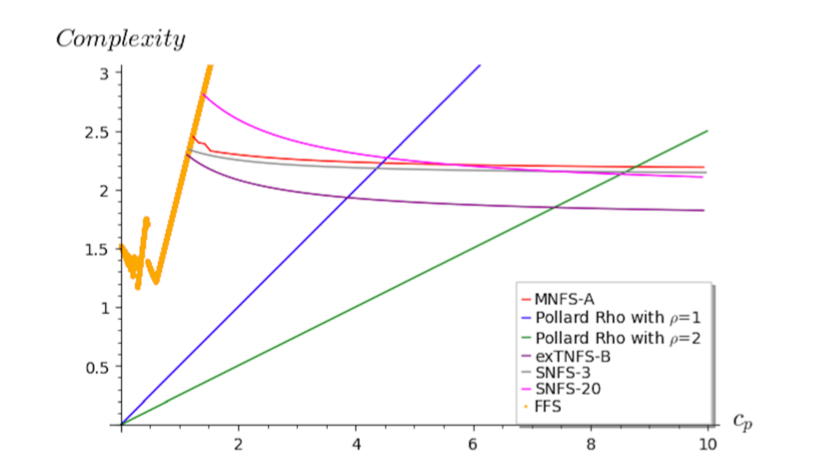## Linear algebra

Linear algebra is a widely used tool both in mathematics and computer science, and cryptography is no exception to this rule. Yet, it introduces some particularities, such as dealing with linear systems that are often sparse, or, in other words, linear systems inside which a lot of coefficients are equal to zero. We propose to enlarge this notion to nearly sparse matrices, caracterized by the concatenation of a sparse matrix and some dense columns, and to design an algorithm to solve this kind of problems. Motivated by discrete logarithms computations on medium and high caracteristic finite fields, the Nearly Sparse Linear Algebra briges the gap between classical dense linear algebra problems and sparse linear algebra ones, for which specific methods have already been established. Our algorithm particularly applies on one of the three phases of the number field sieve which precisely consists in finding a non trivial element of the kernel of a nearly sparse matrix.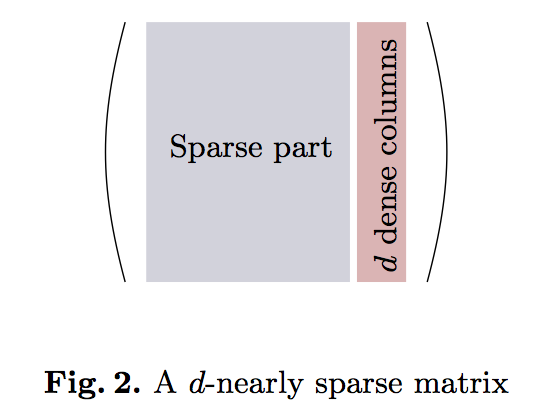## Eclideans lattices, a useful tool

### Side-channel attack on ECDSA

ECDSA is a widely deployed signature protocol based on elliptic curves. Most of the known attacks using side-channels deal with potential weaknesses in the implementation of the scalar multiplication. When this multplication is implemented thanks to the wNAF reprensation, the attacks are divided into two parts: first the gathering of data through side-channels (for instance time measurements on cache access), and then, the processing of this data thanks to Euclidean lattice based methods. Studying in details one of these methods called the Extended Hidden Number Problem (EHNP), we proved how to recover the secret key of ECDSA with only 3 signatures. It was a theoretical bound already known but never achieved in practice. Our method is faster and shows a higher probability of success than most of previous attacks, using the same implementation weakness. In particular, our lattice construction allows to recover the secret key even with a small amount of misread digits during the side-channel lecture of the traces (up to 2% of misread digits).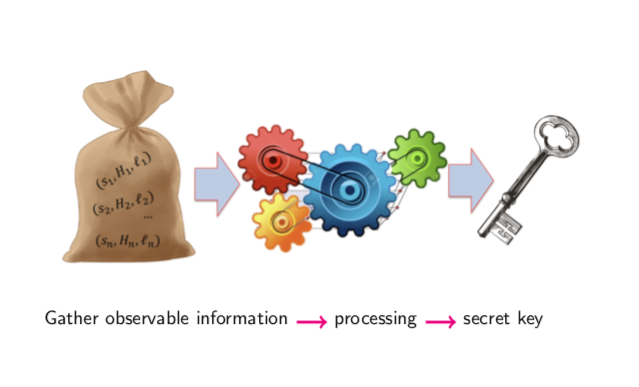### Discrete logarithms lattices, BDD and Minkowski's bound

The question of finding the most efficient way to pack spheres together, for instance oranges, is quite an old problem. Arranging the spheres so that their centers form an Euclidean lattice helps to find solutions. In particular, for arbitrary norms and dimensions, being able to construct dense lattices helps to give good candidats to this problem. However the density cannot be greater than a given number (depending on the norm and dimension only), called Minkowski's bound. A similar problem, the Bounded Distance Decoding problem (BDD), is the following: let's t be a vector space that comes from a vector v of the lattice alterated by an error e (id est t = v + e), find v. Here, Minkowski's bound shows we cannot decode (id est find v) beyond a given radius, whatever the lattice is. Yet it doesn't mean that we are able to decode up to that radius, neither in the general case, nor for very particular lattices.
We propose a concrete family of dense lattices of arbitrary dimension n in which the lattice Bounded Distance Decoding (BDD) problem can be solved in deterministic polynomial time. This construction is directly adapted from the Chor-Rivest cryptosystem (1988). The lattice construction needs discrete logarithm computations that can be made in deterministic polynomial time for well-chosen parameters. Each lattice comes with a deterministic polynomial time decoding algorithm able to decode up to large radius. Namely, we reach decoding radius within O(log n) Minkowski’s bound, for both l1 and l2 norms.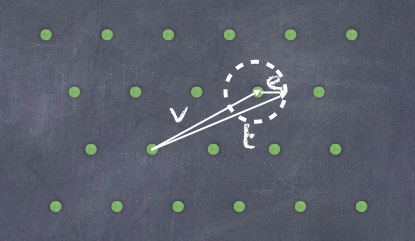## Randomness generation and Bitcoin

Trustworthy generation of public random numbers is necessary for the security of many cryptographic applications. It was suggested to use the inherent unpredictability of blockchains as a source of public randomness. Entropy from the Bitcoin blockchain in particular has been used in lotteries and has been suggested for a number of other applications ranging from smart contracts to election auditing. However, the malleability of the blockchain entropy shows how an adversary could manipulate these random numbers, even with limited computational power and financial budget.
This analysis provides by the way an illustration of generalised Dyck words.